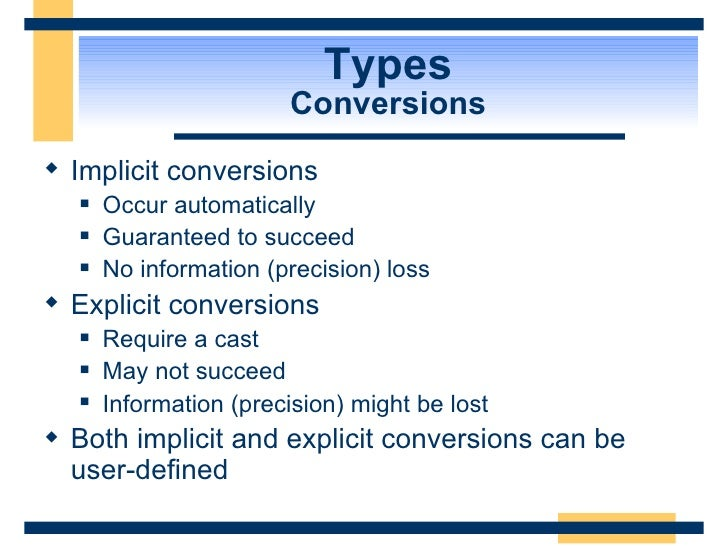# C# Implicit Cast Wrapping for OperatorsPosted in software by Christopher R. Wirz on Wed Sep 28 2016

﻿

Operator overloads allow for conveniences like math and subscripting - but what if you are already given a class from a compiled library? Extension methods must be in static classes, and static classes can't have operator overloads. This means that many times in C# you create wrapper classses just to provide operators. If you are wrapping a library made in C++, this is often done to take advantage of Linq or multi-subscript operators.

In order to allow the methods of the compiled library to accept your newly defined class, you must use the implicit cast operator.

Note: This article does not consider double* op_Subscript(int index), which would result in an unsafe pointer. If going from C++ to C#, it is recommmended to use getters and setters, or properties if there is no subsetting.

Take for example the provided class as follows:

``````
public class Provided {
public Provided(int rows, int columns);
public double GetValue(int row, int column);
public void SetValue(int row, int column, double value);
public void Randomize();
public int Rows {get;}
public int Columns {get;}
}
```
```

This class looks like a Matrix of double values. It's too bad that C++ doesn't support double subscripting; good thing C# can help. While we're at it, let's give the whole thing access as if it were an array. Then, when we add the IEnumerable and IEnumerator interfaces, we can use .Count() and .Sum() methods provided by Linq.

``````
public class Wrapper : IEnumerable<double>, IEnumerator<double>
{
/// <summary>
/// 	Implicit cast from Wrapper to Provided
/// </summary>
/// <param name="x">A Wrapper object</param>
public static implicit operator Provided(Wrapper x)
{
return x._provided;
}

/// <summary>
/// 	Implicit (all Provided can be converted to Wrapper)
///	cast from Provided to Wrapper
/// </summary>
/// <param name="x">A Provided object</param>
public static implicit operator Wrapper(Provided x)
{
return new Wrapper(x);
}

// The private field for the wrapped object

// The private constructor
private Wrapper(Provided obj)
{
_provided = obj;
}

/// <summary>
/// 	Gets the number of values, total
/// </summary>
public int Size => _provided.Rows * _provided.Columns;

/// <summary>
/// 	Sets random values throughout
/// </summary>
public void Randomize() { _provided.Randomize(); }

/// <summary>
/// 	The subscript operatator allows for setting and getting
///	of values given an index (treating the 2-D object like a 1-D array)
/// </summary>
/// <param name="index">The index (0-index)</param>
/// <returns>A double value</returns>
double this[int index]
{
get
{
index = index % Size;
int column = index % _provided.Columns;
int row =  (int)((index - column) / _provided.Columns);
return _provided.GetValue(row, column);
}
set
{
index = index % Size;
int column = index % _provided.Columns;
int row =  (int)((index - column) / _provided.Columns);
_provided.SetValue(row, column, value);
}
}

/// <summary>
/// 	The double subscript operatator allows for setting and getting
///	of values given row and column
/// </summary>
/// <param name="row">The row index (0-index)</param>
/// <param name="column">The column index (0-index)</param>
/// <returns>A double value</returns>
double this[int row, int column]
{
get
{
return _provided.GetValue(row, column);
}
set
{
_provided.SetValue(row, column, value);
}
}

#region IEnumerable

private int _currentIndex = -1;

public double Current => this[_currentIndex];

object IEnumerator.Current => Current;

public IEnumerator<double> GetEnumerator()
{
return this;
}

IEnumerator IEnumerable.GetEnumerator()
{
return this.GetEnumerator();
}

public bool MoveNext()
{
_currentIndex++;
if (_currentIndex < Size)
{
return true;
}
else
{
_currentIndex = -1;
return false;
}
}

public void Reset()
{
_currentIndex = -1;
}
#endregion
}
```
```

Given that implementation and implicit cast, we can now do something like this:

``````
// Make a 5x6 matrix
var p = new Provided(5, 6);
var w = (Wrapper)p;
w.Randomize();
// set a value one way
w[1,3] = 7.2;
// Set the value another way
w = 6.2;
// Get the sum (using Linq)
var sum = w.Sum();
// Get a value from p
var v = p.GetValue(1,3);

// The value v should be 6.2
```
```